### Home > MC2 > Chapter 6 > Lesson 6.2.7 > Problem6-138

6-138.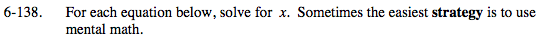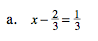Isolate the x variable.

$x-\frac{2}{3}=\frac{1}{3}$

$+\frac{2}{3} \ \ \ +\frac{2}{3}$

x = 1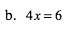What number do you need to divide both sides by to isolate the x variable?

What is 6 divided by 4?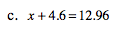Subtract 4.6 from both sides to isolate x.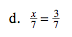Notice that both sides are divided by 7.

x = 3